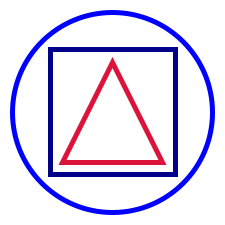# Shapes

## Concept

Constructing simple geometric shapes is provided by the Shape algebra. All the methods on the algebra are constructors, so typically you'd access them by calling methods on the `Picture` object. Here's an example.

``````import doodle.core._
import doodle.java2d._
import doodle.syntax.all._

val basicShapes =
Picture
.circle(200)
.strokeColor(Color.blue)
.on(Picture.square(125).strokeColor(Color.darkBlue))
.on(Picture.triangle(100, 100).strokeColor(Color.crimson))
.strokeWidth(5.0)``````

This program gives the output below.Here is a short description of all the methods provided by `Shape`.

• `empty` creates the empty picture that takes up no space and renders nothing. Useful for that sweet monoid identity and for the base case in recursions.
• `square(sideLength)` creates a square with the given side length.
• `rectangle(width, height)` creates a rectangle with the given width and height.
• `circle(diameter)` creates a circle with the given diameter. Specified in terms of diameter rather than radius so that `square(100)` and `circle(100)` take up the same space.
• `triangle(width, height)` creates an isoceles triangle with the given width and height.

## Implementation

These methods are available on both the `Shape` algebra and `Image`.

Paths→